Lakhmir Singh & Manjit Kaur: Motion, Solutions- 4

# Lakhmir Singh & Manjit Kaur: Motion, Solutions- 4 - Science Class 9

Page No:42

Question 38:
Show by means of graphical method that: v = u + at, where the symbols have their usual meanings.
Solution :
Consider the velocity-time graph of a body shown in figure.
The body has an initial velocity u at a point A and then its velocity changes at a uniform rate from A to B in time t. In other words, there is a uniform acceleration a from A to B, and after time t its final velocity becomes v which is equal to BC in the graph. The time t is represented by OC. To complete the figure, we draw the perpendicular CB from point C, and draw AD parallel to OC. BE is the perpendicular from point B to OE.
Now, Initial velocity of the body, u= OA —–(1)
And,Final velocity of the body, v=BC ——(2)
But from the graph BC =BD + DC
Therefore, v=BD + DC ——(3)
Again DC = OA
So,v =BD + OA
Now, from equation (1), OA =u
So, v=BD + u ——(4)
We should find out the value of BD now. We know the slope of a velocity-time graph is equal to the acceleration, a.
Thus,Acceleration, a= slope of line AB
But AD =OC= t, so putting t in place of AD in the above relation, we get:
or BD=at
Now, putting this value of BD in equation(4), we get:
v= u+ at

consider the velocity - time graph of a body shown in figure: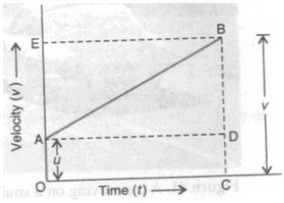the body has an initial velocity u at a point A and then its velocity changes at a uniform rate from A to B  in time t. In other words, there is a uniform acceleration a from A to B, and after time t its final velocity becomes v which is equal to BC in graph. The time t is represented by OC. To complete the figure, we draw the perpendicular CB from point C, and draw AD parallel to OC. BE is the perpendicular from point B to OE.

Now initial velocity of the body, u = OA  --- (1)

and, final velocity of the body, v = BC  --- (2)

But from the graph BC = BD+DC

Therefore v = BD+DC  ----(3)

Again DC = OA

So, v = BD+ OA

Now, from equation  (1), OA = u

So, v= BD+ u  ----(4)

we should find out the value of BD now. We know the slope of the velocity -time graph is equal to the acceleration , a

Thus acceleration a = slope of line AB

But AD = OC= t, so putting t in place of AD in the above relation, we get: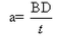or BD = at

Now, Putting the value of BD in equation (4), we get

v= u+at

Question 39:
Show by using the graphical method that: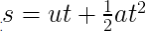where the symbols have their usual meanings.
Solution :
Consider the velocity-time graph of a body shown in figure. The body has an initial velocity u at a point A and then its velocity changes at a uniform rate from A to B in time t. In other words, there is a uniform acceleration a from A to B, and after time t its final velocity becomes v which is equal to BC in the graph. The time t is represented by OC.
Suppose the body travels a distance s in time t. In the figure, the distance travelled by the body is given by the area of the space between the velocity-time graph AB and the time axis OC, which is equal to the area of the figure OABC. Thus:
Distance travelled = Area of figure OABC
= Area of rectangle OADC + area of triangle ABD
Now, we will find out the area of rectangle OADC and area of triangle ABD.
(i) Area of rectangle OADC =OA x OC
= u x t
=ut
(ii) Area of triangle ABD= (1/2)x Area of rectangle AEBD
(1/2) x t x at
(1/2) at2
Distance travelled, s = Area of rectangle OADC + area of triangle ABD

Consider the velocity-time graph of a body shown in figure. The body has an initial velocity u at a point A and then its velocity changes at a uniform  rate from A to B in time t. In other words, there is a uniform acceleration a from A to B, and after time t its final velocity become v which is equal to BC in the graph. The time t is represented by OC.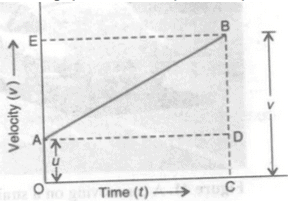Suppose the body travel a distance in time t . in the figure, the distance travelled by the body is given by the area of the space between the velocity-time graph AB and the time axis OC, which is equal to the area of the figure OABC. Thus:

Distance travelled  = Area of figure OABC

= Area of rectangle OADC+ area of triangle ABD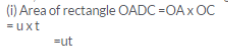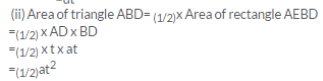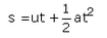Question 40:
Derive the following equation of motion by the graphical method : v2 = u2 + 2as, where the symbols have their usual meanings.
Solution :
Consider the velocity-time graph of a body shown in figure. The body has an initial velocity u at a point A and then its velocity changes at a uniform rate from A to B in time t. In other words, there is a uniform acceleration a from A to B, and after time t its final velocity becomes v which is equal to BC in the graph. The time t is represented by OC. To complete the figure, we draw the perpendicular CB from point C, and draw AD parallel to OC. BE is the perpendicular from point B to OE.
The distance travelled s by a body in time t is given by the area of the figure OABC which is a trapezium.
Distance travelled, s= Area of trapezium OABC
Now, OA + CB = u + v and OC =t Putting these values in the above relation, we get:
Eliminate t from the above equation. This can be done by obtaining the value of t from the first equation of motion.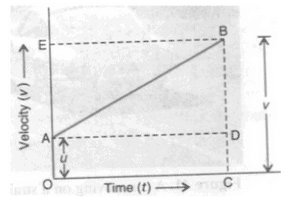Consider the velocity of time graph of a body shown in figure. The body has an initial velocity u at a point A and then its velocity changes at a uniform rate from A to B in time t. In other words, there is a uniform acceleration a from A to B, and after time t its final velocity becomes v which is equal to BC in the graph. the time t is represented by OC. To complete the figure, we draw the perpendicular CB from point C, and draw AD parallel to OC. BE is the perpendicular  from point B to OE

the distance travelled s by a body in time t is given by the area of the figure OABC which is trapezium.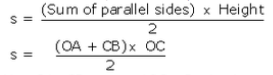Now, OA +CB = u+v and OC = t putting these values in the above relation, we get: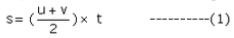Eliminate t from the above equation. This can be done obtaining the value of t from the first equation of motion.

thus v = u +at (first equation of motion)

and at = v

Page No:43

Question 53:
The graph given alongside shows the positions of a body at different times. Calculate the speed of the body as it moves from :
(i) A to B,
(ii) B to C, and
(iii) C to D.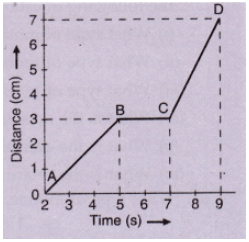Solution :
(i) The distance covered from A to B is( 3-0) =3 cm
Time taken to cover the distance from A to B =(5 -2) =3s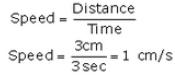(ii) The speed of the body as it moves from B to C is zero.
(iii) The distance covered from C to D is (7-3)=4 cm
Time taken to cover the distance from C to D = (9-7)=2s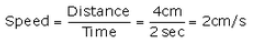Question 54:
What can you say about the motion of a body if:
(a) its displacement-time graph is a straight line ?
(b) its velocity-time graph is a straight line ?
Solution :
(a) The body has a uniform velocity if its displacement-time graph is a straight line.
(b) The body has a uniform acceleration if its velocity-time graph is a straight line.

Question 55:
A body with an initial velocity x moves with a uniform acceleration y. Plot its velocity-time graph.
Solution :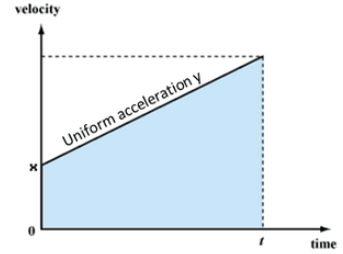Question 56:
Given alongside is the velocity-time graph for a moving body :
Find :
(i) Velocity of the body at point C.
(ii) Acceleration acting on the body between A and
(iii) Acceleration acting on the body between B and C.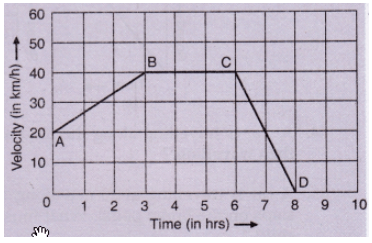Solution :
(i) BC represents uniform velocity. From graph, we see that the velocity of the body at point C = 40km/h
(ii) Acceleration between A and B = slope of line AB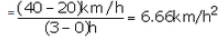(iii) BC represents uniform velocity, so acceleration acting on the body between B and C is zero.

Question 57:
A body is moving uniformly in a straight line with a velocity of 5 m/s. Find graphically the distance covered by it in 5 seconds.
Solution :
Distance travelled = Area of rectangle OABC
= OA x OC
= 5 x 5 =25 m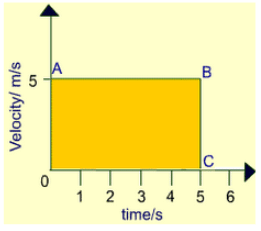Question 58:
The speed-time graph of an ascending passenger lift is given alongside.
What is the acceleration of the lift:
(i) during the first two seconds ?
(ii) between second and tenth second ?
(iii) during the last two seconds ?

Solution:

(i) Acceleration during first two seconds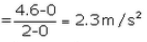(ii) Acceleration between second and tenth second is zero, since the velocity is constant during this time.

(iii) Acceleration during last two seconds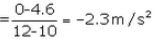Question 59:
A car is moving on a straight road with uniform acceleration. The speed of the car varies with time as follows :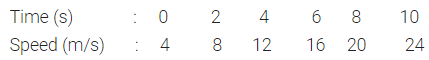Draw the speed-time graph by choosing a convenient scale. From this graph :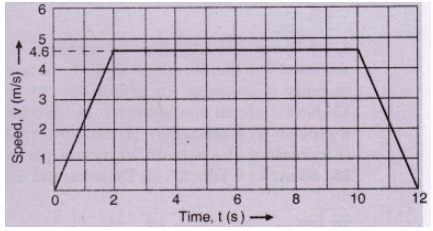(i) Calculate the acceleration of the car.
(ii) Calculate the distance travelled by the car in 10 seconds.
Solution :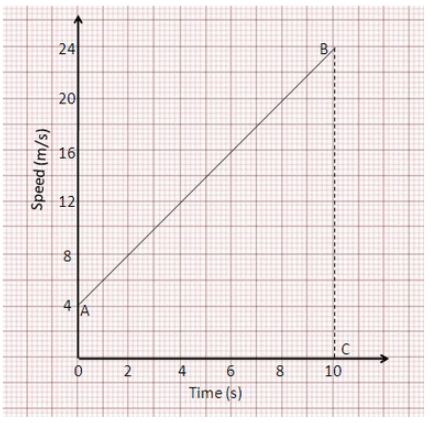(i) Acceleration of the car = slope of line AB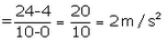(ii) Distance travelled by the car in 10 s = area of trapezium OABC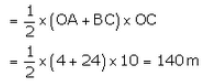Question 60:
The graph given alongside shows how the speed of a car changes with time: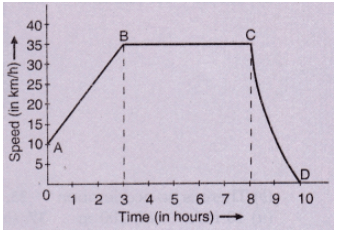(i) What is the initial speed of the car ?
(ii) What is the maximum speed attained by the car ?
(iii) Which part of the graph shows zero acceleration ?
(iv) Which part of the graph shows varying retardation ?
(v) Find the distance travelled in first 8 hours.
Solution :
(i) Initial speed of the car=10km/h
(ii) Maximum speed attained by the car= 35km/h
(iii) BC represents zero acceleration.
(iv) CD represents varying retardation.
(v)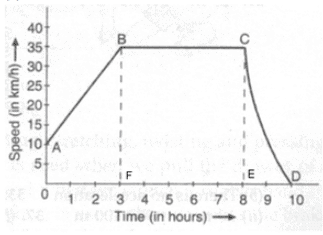Distance travelled in first 8 hrs:

s= Area of trapezium OABF + Area of rectangle BCEF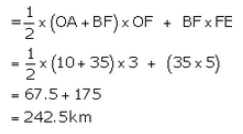Question 61:
Three speed-time graphs are given below :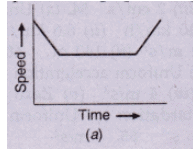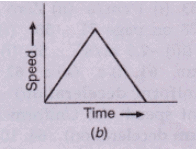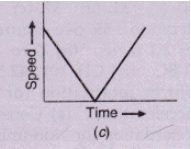Which graph represents the case of:
(i) a cricket ball thrown vertically upwards and returning to the hands of the thrower ?
(ii) a trolley decelerating to a constant speed and then accelerating uniformly ?
Solution :
(i) Graph (c): The speedof the ball goes on decreasing uniformly as it moves upward, reaches zero at the highest point, and then increases uniformly as it moves downward.
(ii) Grap(a): The speed of the trolley decreases uniformly, then it moves at a constant speed, and then the speed increases uniformly.

Page No:44

Question 62:
Study the speed-time graph of a car given alongside and answer the following questions: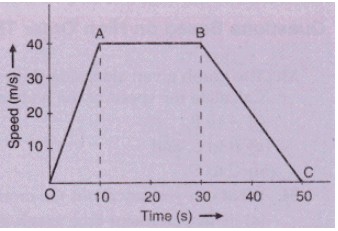(i) What type of motion is represented by OA ?
(ii) What type of motion is represented by AB ?
(iii) What type of motion is represented by BC ?
(iv) What is the acceleration of car from O to A ?
(v)What is the acceleration of car from A to B ?
(vi) What is the retardation of car from B to C ?
Solution :
(i) OA represents uniform acceleration
(ii) AB represents constant speed.
(iii) BC represents uniform retardation.
(iv) Acceleration of car from O to A = slope of line OA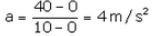(v) Acceleration of car from A to B is zero as it has uniform speed during this time.
(vi) Retardation of car from B to C = slope of line BC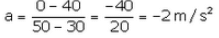Question 63:
What type of motion is represented by each one of the following graphs ?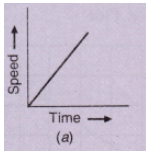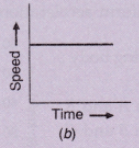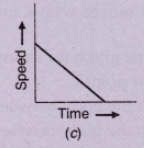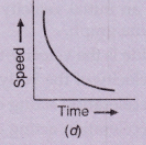Solution :
(i) Graph (a) represents uniform acceleration.
(ii) Graph (b) represents constant speed.
(iii) Graph (c) represents uniform retardation.
(iv) Graph (d) represents non-uniform retardation.

Question 64:
A car is travelling along the road at 8 ms-1. It accelerates at 1 ms-2 for a distance of 18 m. How fast is it then travelling ?
Solution :
Initial velocity, u=8m/s
Acceleration, a=1m/s2
Distance, s=18m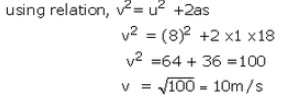Question 65:
A car is travelling at 20 m/s along a road. A child runs out into the road 50 m ahead and the car driver steps on the brake pedal. What must the car’s deceleration be if the car is to stop just before it reaches the child ?
Solution :
Initial velocity, u=20m/s
Final velocity, v=0m/s
Distance, s=50m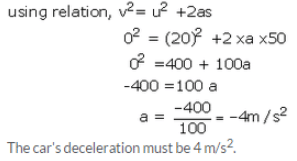The document Lakhmir Singh & Manjit Kaur: Motion, Solutions- 4 | Science Class 9 is a part of the Class 9 Course Science Class 9.
All you need of Class 9 at this link: Class 9

## Science Class 9

66 videos|355 docs|97 tests

## FAQs on Lakhmir Singh & Manjit Kaur: Motion, Solutions- 4 - Science Class 9

 1. What is motion in physics?Ans. Motion in physics refers to the change in position of an object with respect to its surroundings over time. It involves the concepts of distance, displacement, speed, velocity, and acceleration.
 2. What are the different types of motion?Ans. There are several types of motion, including linear motion, circular motion, periodic motion, and rotational motion. Linear motion occurs when an object moves along a straight line, while circular motion involves movement along a circular path. Periodic motion is the repetitive motion of an object, such as the motion of a pendulum. Rotational motion occurs when an object spins or rotates around a fixed axis.
 3. How is speed different from velocity?Ans. Speed is a scalar quantity that measures how fast an object is moving, regardless of its direction. It is calculated by dividing the distance traveled by the time taken. On the other hand, velocity is a vector quantity that specifies both the speed and direction of an object's motion. It is calculated by dividing the displacement of an object by the time taken.
 4. What is the difference between distance and displacement?Ans. Distance is the total length of the path traveled by an object, regardless of its direction. It is a scalar quantity and is always positive. Displacement, on the other hand, is the change in position of an object from its initial position to its final position in a specific direction. It is a vector quantity and can be positive, negative, or zero, depending on the direction of motion.
 5. How does acceleration affect motion?Ans. Acceleration is the rate at which an object's velocity changes over time. If an object undergoes acceleration, it means that its speed, direction, or both are changing. Acceleration can either be positive, indicating an increase in velocity, or negative, indicating a decrease in velocity. A higher acceleration will result in a quicker change in velocity, leading to a more rapid change in motion.

## Science Class 9

66 videos|355 docs|97 testsExplore Courses for Class 9 examSignup to see your scores go up within 7 days! Learn & Practice with 1000+ FREE Notes, Videos & Tests.
10M+ students study on EduRev
Track your progress, build streaks, highlight & save important lessons and more!
Related Searches

,

,

,

,

,

,

,

,

,

,

,

,

,

,

,

,

,

,

,

,

,

,

,

,

;